Question

If the random variable z is the standard normal score and a >0 is it true that p ( z >a ) = p ( z < a ) ? Why pi r Why or What not?

yes because normal distribution means is 0 and variance is 1.
What is the property does the distribution have?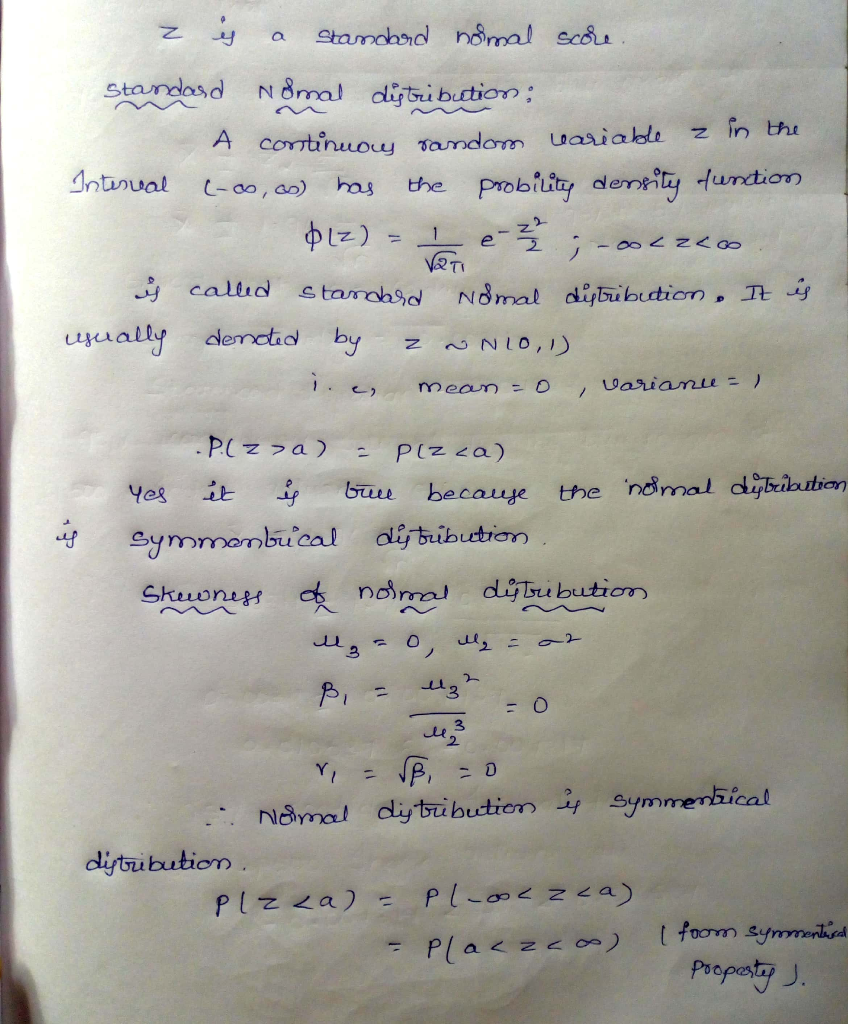#### Earn Coins

Coins can be redeemed for fabulous gifts.

Similar Homework Help Questions
• ### If a random variable z is the standard normal score, then the standard deviation of the distribution is 1

1. If a random variable z is the standard normal score, then the standard deviation of the distribution is 1. ____ T/F

• ### Let z be a normal random variable with mean 0 and standard deviation 1. What is...

Let z be a normal random variable with mean 0 and standard deviation 1. What is P(z > -1.1)? -0.8643 0.8643 -0.1357 0.1357 If all possible random samples of size n are taken from a population that is not normally distributed, and the mean of each sample is determined, what can you say about the sampling distribution of sample means? It is approximately normal provided that n is large enough. It is positively skewed. It is negatively skewed. None of...

• ### Let z be a random variable with a standard normal distribution. Find P(0 ≤ z ≤...

Let z be a random variable with a standard normal distribution. Find P(0 ≤ z ≤ 0.40), and shade the corresponding area under the standard normal curve. (Use 4 decimal places.)

• ### Recall from class that the standard normal random variable, Z, with mean of 0 and stan- dard devi...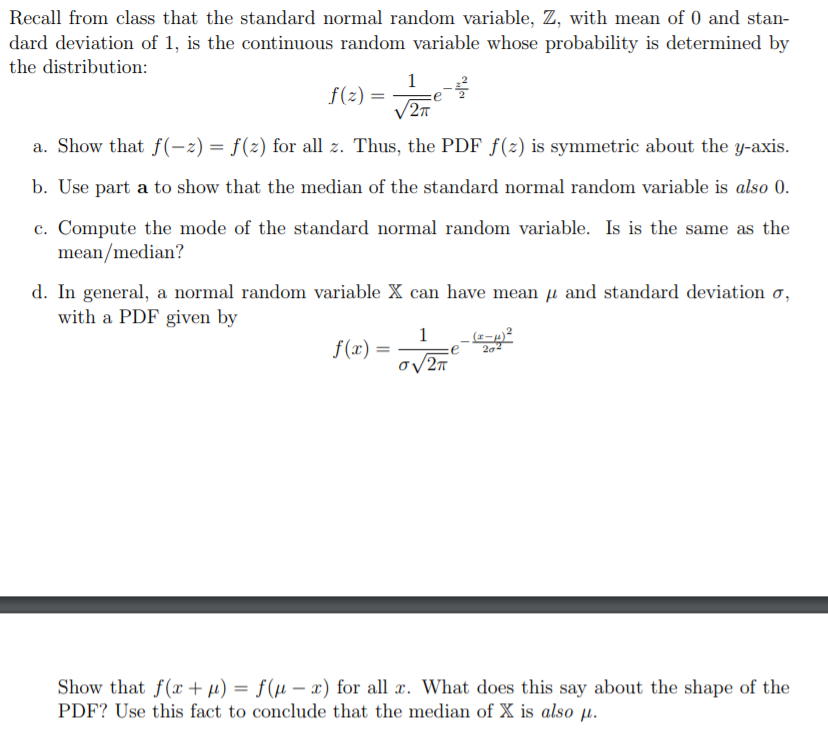Recall from class that the standard normal random variable, Z, with mean of 0 and stan- dard deviation of 1, is the continuous random variable whose probability is determined by the distribution: a. Show that f(-2)-f(2) for all z. Thus, the PDF f(2) is symmetric about the y-axis. b. Use part a to show that the median of the standard normal random variable is also 0 c. Compute the mode of the standard normal random variable. Is is the same...

• ### 1. (5 points) Suppose Z is a random variable that follows the standard normal distribution. a)...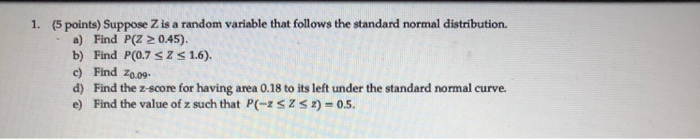1. (5 points) Suppose Z is a random variable that follows the standard normal distribution. a) Find P(Z > 0.45). b) Find P(0.7 SZ 1.6). c) Find 20.09. d) Find the Z-score for having area 0.18 to its left under the standard normal curve. e) Find the value of z such that P(-2SZS2) -0.5.

• ### 1. (5 points) Suppose Z is a random variable that follows the standard normal distribution. a)...1. (5 points) Suppose Z is a random variable that follows the standard normal distribution. a) Find P(Z > 0.45). b) Find P(0.7 SZ 1.6). c) Find 20.09. d) Find the Z-score for having area 0.18 to its left under the standard normal curve. e) Find the value of z such that P(-2SZS2) -0.5. 3. (4 points) The scores on a test are normally distributed with a mean of 75 and a standard deviation of 8. a) Find the proportion...

• ### Assume Z is a random variable with a standard normal distribution and c is a positive...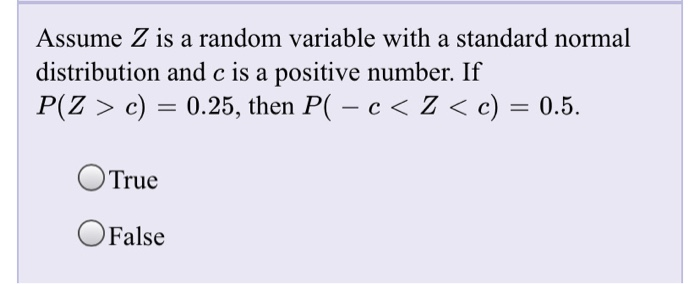Assume Z is a random variable with a standard normal distribution and c is a positive number. If P(Z > c) = 0.25, then PC – c< < c) = 0.5. O True OFalse Exactly 50% of the area under the normal curve lies to the left of the mean. O True OFalse If X represents a random variable coming from a normal distribution and P(X < 5.2) = 0.5, then P(X > 5.2) = 0.5. O True O False

• ### 40 B 1. (5 points) Suppose Z is a random variable that follows the standard normal...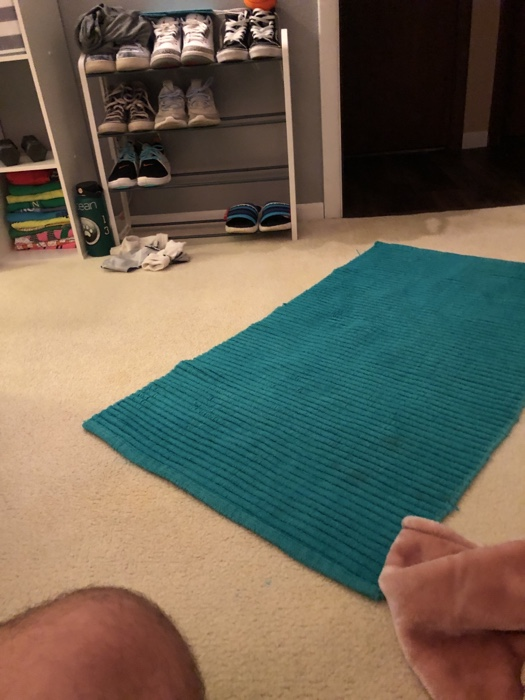40 B 1. (5 points) Suppose Z is a random variable that follows the standard normal distribution. a) Find P(Z > 0.45). b) Find P(0.7 SZ 1.6). c) Find 20.09. d) Find the Z-score for having area 0.18 to its left under the standard normal curve. e) Find the value of z such that P(-2SZS2) -0.5.

• ### Given that z is a standard normal random variable, find the z-score for a situation where...

Given that z is a standard normal random variable, find the z-score for a situation where the area to the left of z is 0.0901.

• ### Given that z is a standard normal random variable, find the z-score for a situation where...

Given that z is a standard normal random variable, find the z-score for a situation where the area to the left if z is 0.8907.